# Fractions + reason - math problems

#### Number of problems found: 82

• Cake fractions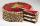Thomas ate 1/3 of cake, Bohouš of the rest of the cake ate 2/5. What fraction of cake left over for others?
• Wage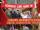Increasing the minimum wage while maintaining the other parameters of the system, unemployment will be:
• Unknown number 23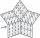Find 2/3 of unknown number, which is two thirds of the 99.
• MidnightHow many hours are, if the time that elapsed since 8:00 is 2/5 of the time that will past till midnight?
• Coin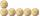How many 50-cent coins must be put in a row that next 50-cent coins after them (above) rolled on it axis?
• PipeSteel pipe has a length 2.5 meters. About how many decimetres is 1/3 less than 4/8 of this steel pipe?
• Unknown numberFind the unknown number equal to a quarter of a fifth of a number, which is by 152 more than an unknown number.
• DiscountThe dress was discounted by CZK 115, which was 12% of the original price. What is the cost of the dress after the discount?
• Fuel consumption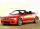Škoda car has an average diesel consumption of 5.9 liters per 100 kilometers. What is the car range for a full tank of volume 70 liters?
• Clock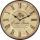What time is now if the time elapsed afternoon is 2/10 of time that elapses before midnight?
• Grandmother's clocks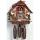Grandmother's clock is late every hour by half a minute. Grandmother set the clock exactly at 8.00 am. How many hours will show after 24 hours?
• A bagA bag contains 9 blue marbles and 1 green marble. What is the probability of drawing a blue marble followed by a green marble, without replacing the first marble before drawing the second marble.
• TV failThe TV has after 10,000 hours average 35 failures. Determine the probability of TV failure after 400 hours of operation.
• BonusThe gross wage was 1323 USD including 25% bonus. How many USD were bonuses?
• DoublingThe message is spreading that each day has doubled the number of people who know about it. All know a message for 20 days. How long known it, eighth people?
• Reminder and quotientThere are given the number C = 281, D = 201. Find the highest natural number S so that the C:S, D:S are with the remainder of 1,
• Pocket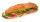Mirka spent on a trip for gifts half pocket. For the third remain money bought a bagel. Six euros left her. How many euros had Mirka in pocket?
• Marriage sttusIn our city, there are 3/5 of the women married to 2/3 of the men. Find what part of the population is free.
• Competition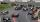The organizers of competion wanted to spent big amount of money to pay for the competitors. One third of this amount spent on prizes and the remaining € 5,000 to diplomas. How much was committed for rewards?
• On a farm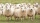On a farm, there are 90 rabbits, 700 sheep, 300 cattle and 500 pigs. What percentage of the total number of animals are rabbits?

Do you have an interesting mathematical word problem that you can't solve it? Submit a math problem, and we can try to solve it.

We will send a solution to your e-mail address. Solved examples are also published here. Please enter the e-mail correctly and check whether you don't have a full mailbox.

Please do not submit problems from current active competitions such as Mathematical Olympiad, correspondence seminars etc...

Need help to calculate sum, simplify or multiply fractions? Try our fraction calculator. Reason - math problems. Fraction Word Problems.